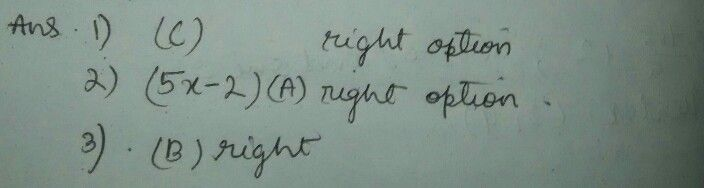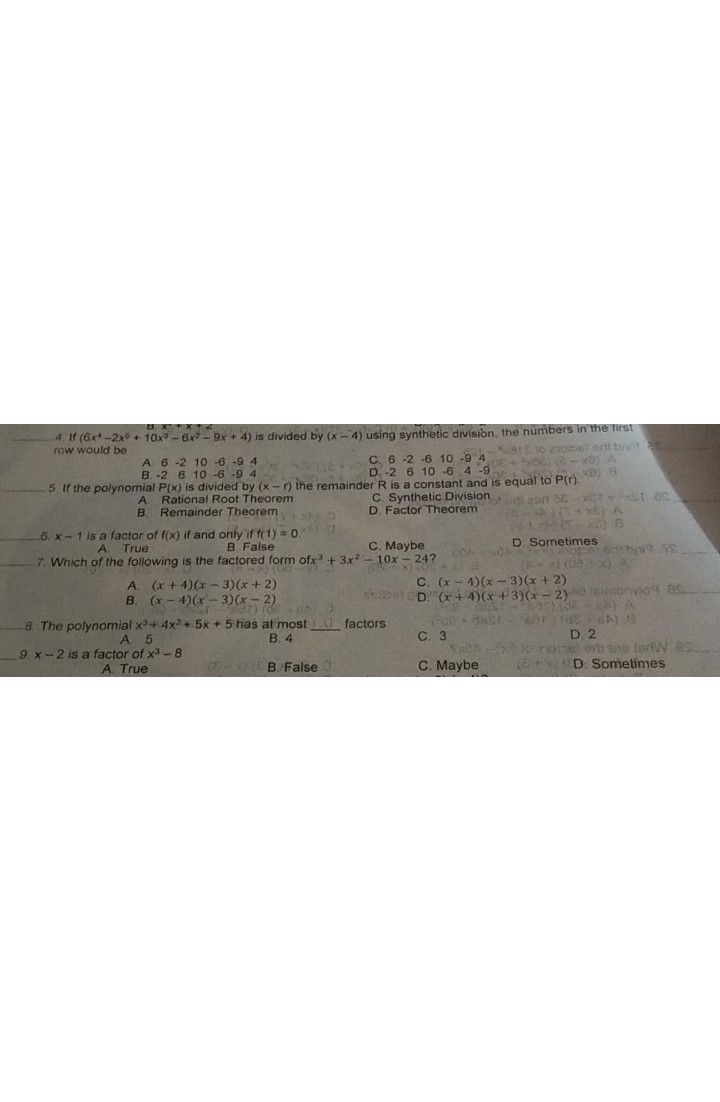Symbol
ProblemGoley ed as torw 1. What is the $om0andor3$ $10x$ $10x$ - 8 4 - A. $x^{2}+x+2$ -4 C -4 4et o bnia ss B. $5x-2$ YS ea D. 0 2. Which is the divisor? A. $5x-2$ D. $5x^{3+3x^{2+8x-}}$ $0$ 8 B. $x^{2}+x+2$ E e A 3. What is the quotient? D. C. $x^{2}+x-2$ $-x^{2}+x+2$ to e1olbet erli bnA MS $A$ $x^{2}-x+2$ $x^{2}+x+2$ $4.1r\left(6x^{4}-2x^{5}+1^{B}$ $0x^{3}-6x^{2}-9x+4\right)$ is divided by $\left(x-4\right)$ using synthetic division, the numbers in the first row would be ed bro 2 $A$ 6 -2 10 --6 6 --9 9 4 4 C. 6 -2 -6 $10-9$ 4 a) A B. -2 6 10 D. -2 6 $10-6$ 4 -9 5. If the polynomial $P\left(x\right)1s$ divided by $\left(x-r\right)$ the remainder R is a constant and is equal to $P\left(r\right)$ A. Rational Root Theorem $C.$ Synthetic Division B. Remainder Theorem D. Factor Theoremo orti eed 2e (+ x) (-
10th-13th grade
Other
Search count: 105
SolutionQanda teacher - amrit69Student
thank youQanda teacher - amrit69
please evaluate with some gifts we need your appreciationStudent